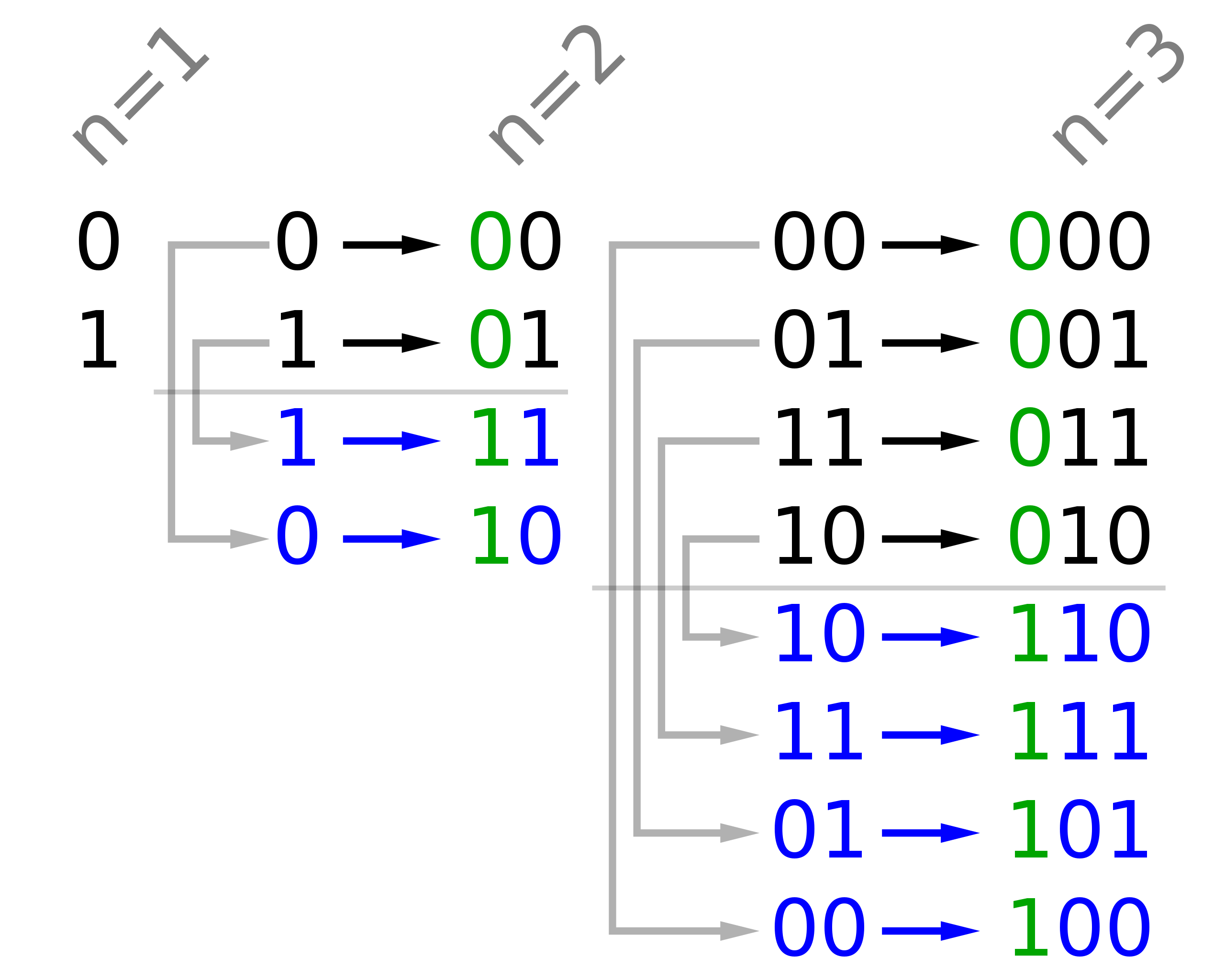# [LeetCode] 89. Gray Code

An n-bit gray code sequence is a sequence of `2n` integers where:

• Every integer is in the inclusive range `[0, 2n - 1]`,
• The first integer is `0`,
• An integer appears no more than once in the sequence,
• The binary representation of every pair of adjacent integers differs by exactly one bit, and
• The binary representation of the first and last integers differs by exactly one bit.

Given an integer `n`, return any valid n-bit gray code sequence.

Example 1:

```Input: n = 2
Output: [0,1,3,2]
Explanation:
The binary representation of [0,1,3,2] is [00,01,11,10].
- 00 and 01 differ by one bit
- 01 and 11 differ by one bit
- 11 and 10 differ by one bit
- 10 and 00 differ by one bit
[0,2,3,1] is also a valid gray code sequence, whose binary representation is [00,10,11,01].
- 00 and 10 differ by one bit
- 10 and 11 differ by one bit
- 11 and 01 differ by one bit
- 01 and 00 differ by one bit
```

Example 2:

```Input: n = 1
Output: [0,1]```

Constraints:

• `1 <= n <= 16`

00 - 0
01 - 1
11 - 3
10 - 2

00 - 0
10 - 2
11 - 3
01 - 1Java实现

``` 1 class Solution {
2     private List<List<Integer>> dp;
3
4     public List<Integer> grayCode(int n) {
5         dp = new ArrayList<>(n + 1);
6         for (int i = 0; i <= n; i++) {
8         }
9         // 1. dp初始状态，即把数字0的格雷码先加入dp中
11         // 2. dp递推过程，通过循环依次计算数字1, 2, 3, ..., n 的格雷码
12         for (int num = 1; num <= n; num++) {
13             // 数字num的格雷码的总个数 2^num
14             int count = 1 << num;
15             for (int i = 0; i < count; i++) {
16                 int value;
17                 if (i < count / 2) {
18                     // 前半部分直接复制num - 1的格雷码
19                     value = dp.get(num - 1).get(i);
20                 } else {
21                     // 后半部分通过在首位添加1位1得到
22                     int add = 1 << (num - 1);
23                     value = dp.get(num).get(count - 1 - i) + add;
24                 }
26             }
27         }
28         return dp.get(n);
29     }
30 }```

LeetCode 题目总结

[LeetCode] 89. Gray Code

(0)
(0)